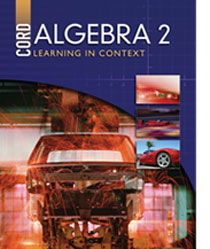# Algebra 2 - First Edition

Some links are repeated for use with more than one lesson.

### 6.1 Solving Quadratic Equations by Graphing

http://www.teachertube.com/viewVideo.php?video_id=20844

http://www.ronblond.com/M11/QFA.sf.APPLET/index.html
NEW This page contains an applet for graphing quadratic equations and see the solution. This website requires Java to run some of its features.

### 6.3 Solving Quadratic Equations by Completing the Square

http://www.freemathhelp.com/Lessons/Algebra_2_Completing_the_Square_

This page has a computer generated lecture and notes on completing the square and the quadratic formula.

This page contains a tutorial on completing the square and shows how the quadratic formula is derived.

### 6.4 Solving Quadratic Equations by Factoring

http://www.regentsprep.org/regents/math/algtrig/ATE3/indexATE3.htm